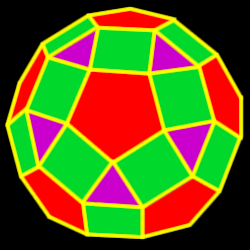# Rhombicosidodecahedron

Properties of the rhombicodidodecahedron: Number of faces, edges and dihedral angle measure

The rhombicosidodecahedron is created by either truncating (cutting off) the dodecahedron one half of the way into each side
or by truncating the icosahedron one half of the way into each side62 total faces:
20 equilateral triangles, 30 squares and 12 regular pentagons

60 vertices:
1 triangle, 2 squares and 1 pentagon

120 edges

Dihedral angles:
148 degrees, 17 minutes for the pent-sqr angle and 159 degrees, 6 minutes for the tri-sqr angle